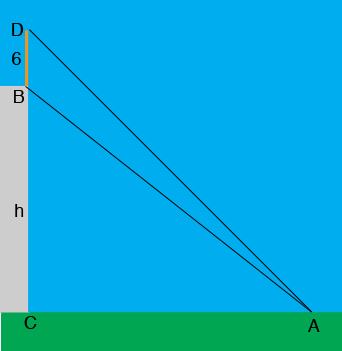SEARCH HOMEMath Central Quandaries & QueriesQuestion from prateek, a student: the height of a flagpole on top of a building is 6m. the angle of elevation of the top and bottom of the flagpole are 45 and 30 respectively. find the height of the buildingHi,

Here is my diagram,The measure of the angle CAD is $45^o$ and angle DCA is a right angle. What is the measure of the angle ADC? The length of DC is h + 6 meters. What is the length of the side CA?

The measure of the angle CAB is $30^o$. What is the measure of the angle ABC? The length of BC is h meters. What does the triangle ABC tell you about the length of CA.

Solve for h.

PennyMath Central is supported by the University of Regina and the Imperial Oil Foundation.